# 1 Introduction

The physicist and mathematician Edward Witten (of M theory renown and I’m sure among other contributions) recently published to arXiv.org a paper serving as a mini-introduction to information theory, including both classical and quantum considerations: A Mini-Introduction To Information Theory [arXiv:1805.11965], which I am currently reading through (although the paper is only 39 pages, the information density is such that intentional review takes a bit more time and effort than a newspaper article might for instance). Although I have had some extracurricular interest in the field for a small while (including auditing a series of MOOC’s on eDx.org), I find that there are still several mathematical notations and conventions (especially in the quantum cases) that are common obstacles to comprehension, many of which these type of papers take for granted in a reader, thus I find myself often turning to supplemental resources such as wikipedia.org, mathworld.wolfram.com, or google.com as I work my way through these type of papers. Since am going through the effort anyway, I figured it might be of potential benefit to others new to the field to document a few of these points I come across along the way, which will be the purpose of this essay. Note that this won’t be a comprehensive review of all of the notations and principles included in the paper, this is merely meant to capture a few of the points that I suspect may be less intuitive to someone with the starting point of an engineering degree or the like. As a means of organizing this presentation, I’ll follow the lead of Witten’s paper and present points in the order that they appear in the Mini-Introduction including use of that paper’s section and equation numbering convention, here again is the arXiv: A Mini-Introduction To Information Theory [arXiv:1805.11965]. I’ll more closely follow the narrative of the paper in the classical section and then as we get into the quantum aspects I expect will transition more to supplemental takes. This essay is intended as a supplemental resource, and a reader looking to get the full value here should consider reading it either in parallel or adjacent to a review of Witten’s work.

# 2 Classical Information Theory

It is certainly appropriate that this paper begins with an overview of Shannon entropy. Claude Shannon’s paper A Mathematical Theory of Computation served as the origination of the field of information theory and everything that follows here is built off of that foundation. The core idea of Shannon entropy is that given a signal (such as for example a binary code’s series of 1’s and 0’s or alternatively e.g. a textual communication derived from a series of letters grouped into words and sentences), it is possible to measure the information content of that passage based on other features we can derive from the signal. For example, just from inspecting a signal we can determine the range of potential characters (such as a binary code’s two states 0 and 1 or the English alphabet’s 26 letters), and we can also measure the frequency of occurrence for each state and thus derive the probability of occurrence for each signal (for example in an English passage there will be a higher frequency of vowels than consonants). Using just these two features, it is possible to derive an entropy value S for each additional point in a signal.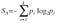Eq 2.7, the general definition for Shannon Entropy S, here p is the probability of occurrence for a given point i in a range of k possible points (such as k=2 for binary code) of distribution A. Note the convention of log base 2, we will abbreviate this to just “log” going forward.

It is further demonstrated that this entropy is constrained to S >= 0, where 0 would correspond to Shannon entropy of a message with complete certainty (such as a unitary code of all 1’s). The S value tells us a few things, a key one being that for a signal of length N points, we can derive the number of equivalent bits of information we can extract from a message — or another way of looking at this is number of bits for maximum compression of a signal.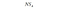Eq 2.8, a formula to derive the equivalent number of bits that can be extracted from a signal of length N with points of distribution A and Shannon Entropy S.

Note that this derivation of limits assume no correlation between points in a signal, this is a simplification and thus there is actually potential for further improvement given further knowledge (such as knowing the rules of grammar for an English passage), although one that would be washed out as N gets sufficiently large.

The usefulness of this Shannon entropy measure becomes more apparent as we extend applications to two-party communication. As is a common convention in the field of information science, we’ll consider two illustrative parties Alice and Bob, where Alice is trying to communicate some message based on a signal distribution X, and Bob receiving a resulting signal with distribution Y, where the difference between the two distributions X and Y originates from such interferences as environmental noise or other errors of signal transmission. In a classical message using binary code transmissions these errors would manifest as bit flips on random points (meaning a point intended as 0 would be read as a 1 or vice versa), in a quantum communication there would be other potential channels of error such as bit flips, phase flips, depolarizing, amplitude dampening, or phase dampening — more on quantum channels to come.

In analysis of this communication, we can evaluate the entropy across a few different bases. The entropy S of distribution X (we’ll call SX) we already know how to derive, just as we know how to derive SY. Another basis that we can consider for this system is SXY, which represents the entropy for the joint system of X and Y, of which the probability p(xi,yj) used in the derivation represents the joint probability of experiencing points x(i) from Alice’s distribution X and y(j) from Bob’s distribution Y.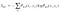(via Eq 2.14) — A formula to demonstrate the entropy derivation for the joint distributions X and Y.

The conditional entropy comes into play when we want to try to see through Bob’s eyes. Bob doesn’t know with certainty Alice’s original message, but we will assume that he has an idea of Alice’s signal distribution X. Thus when he receives a signal point y(j) from his distribution Y, a reasonable question for him to ask himself is “What is the probability that Alice sent the point x(i) given that I received the point y(j)?” This conditional probability can then be used to derive the conditional entropy for this state of affairs (note the constraint that this conditional entropy be >= 0, an interesting bit of foreshadowing is laid from Witten’s comment that this is not necessarily true for a quantum signal).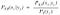Eq 2.12 — The conditional probability that Alice sent x(i) given that Bob receives y(j).(via Eq’s 2.14 and 2.15) — A formula for Bob to calculate conditional entropy, assuming he receives points y(j) from distribution Y and know’s Alice’s distribution X but not with certainty the contents of her signal x(i).

I’m not adding much to the discussion beyond what Witten has laid out by including the following, but it is an important point of the narrative so will go ahead and include anyway, as part of the goal here is also to highlight key passages in the paper. The information about X that Bob gains from what he sees in signal Y is called the mutual information.Eq 2.16 — The information about X that Bob gains from what he sees in signal Y is called the mutual information.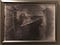Image from the first photograph, 1826.

This section of the paper is devoted to a proof that this mutual information figure is nonnegative ( I(X;Y) >= 0 ), derived by first introducing the concept of relative entropy. Consider the case where we believe a distribution X is described as Q, but this Q is just a hypothesis, one we need to test to confirm. Let’s further say that the actual distribution X is described as P, and so there is a difference between our hypothesis for distribution Q and the correct distribution P of X. The more measurements we take of X (of quantity N), the more information we can infer about the difference between the correct distribution P and our hypothesis distribution Q. Based on our N measurements of X, we can infer that the probability that our hypothesis Q = P (the correct distribution) converges to the following formula for sufficiently large N:(via Eq’s 2.19 and 2.20) The probability that for distribution X our hypothesis distribution Q = P (the correct distribution) for sufficiently large number N measurements of X, where qi is the Q derived probability of a measurement producing the measured point xi and pi is the actual probability of a measurement producing the measured point xi.

The entropy included in the exponent of this function is the relative entropy, also known as the Kullback-Liebler divergence, which from the derivation we know is nonnegative.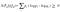(via Eq 2.20) — The relative entropy between our hypothesis distribution Q and the actual distribution P — aka the Kullback-Liebler divergence.

We’ll find that if our hypothesis distribution is correct (P = Q) then the Kullback-Liebler will be ~ 0 (with more certainty for increasing values of N), and if our hypothesis distribution is incorrect our inferred probability from Eq 2.19 will shrink with sufficient N.

The proof that the mutual information (Eq 2.16) is nonnegative is developed by extending this relative entropy from the distribution X to an equivalent joint distribution X and Y, and using some logarithmic properties to show that the relative entropy of this joint distribution is equivalent to the mutual information between these two distributions, which must therefore also be nonnegative.

We made an interesting leap by building on the equivalence between the relative entropy for a single distribution X or an associatively derived joint distribution X,Y. It’s probably worth a clarification that the X in the joint distribution wasn’t meant to be equivalent to the X from the single distribution — it may be easier to think of the equivalent single distribution as X’, where for each case the probability p(x’) = p(xi,yj).

The considerations of joint probability distributions are more in play in this section, as we start to consider what happens when we can make observations for only one of the distributions (X) but not of the other (Y). One thing we can infer off the bat for this case is that adding observations to Y can only help our ability to infer the differences between our hypothesis distribution Q(X,Y) and the actual distribution P(X,Y), thus we can make this statement about the relative entropy for the joint distributions X and Y.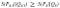Eq 2.28 — This property is called the monotonicity of relative entropy, as observations of Y will only help our ability to infer differences between our hypothesis distribution Q(X,Y) and actual distribution P(X,Y).

We can extend these questions of joint distributions from the pair X,Y to the joint of three distributions X, Y, and Z. It’s easy to replace the Y in equation 2.28 above to an associatively derived joint distribution Y’,Z to give the same result for three distributions. Witten derives a further property of interest for this case using relations between relative entropy and mutual information called strong subadditivity, one that I did not find extremely intuitive. Some further foreshadowing was provided in the comment that this turns out to be true in quantum mechanics — Witten literally describes this finding for the quantum realm as a miracle.

Similarly to the monotonicity of relative entropy as described above, in the mutual information space, what one learns about a random variable X by observing both Y and Z is at least as much as one could learn by observing Y only.

Some closing thoughts on this, the final portion of the classical information theory section of the paper. I think I followed Witten’s narrative in this writeup much closer than I had originally intended. The goal is not to duplicate Witten’s findings, ideally this essay should serve to elaborate on points I found helped with comprehension that Witten might take for granted, while also highlighting some of the more key findings. I’ll try to stick to this philosophy a little closer in the next sections addressing quantum information theory.

# 3 Quantum Information Theory: Basic Ingredients

So rather than trying to follow along completely with the narrative, I’ll begin now trying to offer explanations of key concepts and notations that the paper might take for granted. Quantum mechanic notations I think can be thought of mathematically as linear algebra applied to matrices subject to some constraints describing an analogous property to probability of some outcomes upon measurement — but with the probability expanded to allow for +/- values as well as real and imaginary components (a complex scalar). As the number of potential outcomes incorporated into the analysis grows so does the dimensionality which can be described by a Hilbert space vector of dimensionality. We can incorporate or combine different frames of reference using a tensor product operation. The states are typically represented using the Bra-Ket notation <Bra| and |Ket>, where <Bra| represents a row vector and |Ket> a column vector. We have the ability to translate from a Bra to a Ket vector and vice versa using a conjugate-transpose operation (basically a transpose operation coupled with a complex conjugation that multiplies imaginary portion of scalars by (-1)). I am not positive but assume BraKet is an attempt at a pun on the term bracket which if so I fully support. We can combine the vectors using either an inner product (dot product) abbreviated as <Bra|Ket> or an outer product (tensor product) abbreviated as |Ket><Bra|.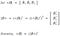Demonstration of conversion between Bra and Ket vectors using the conjugate-transpose operation (basically a transpose operation coupled with a complex conjugation that multiplies imaginary portion of complex scalars by (-1)).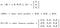Demonstration of Bra Ket vector operations

This BraKet convention can be extended to incorporate an intermediate square matrix in the inner product using standard matrix multiplication, such as: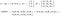Demonstration of Bra Ket inner product incorporating an intermediate square matrix, note that the output is still a complex scalar even with the incorporation of the intermediate matrix.

The tensor product operation (⊗) can also be extended from vectors to matrices input such as: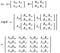Demonstration of tensor product operation applied to matrices. Note these matrices don’t have to be square.

The paper makes use of this operation to demonstrate that if we have two Hilbert spaces of vector dimensions A(i,j) and B(k,l) we can combine the two spaces via tensor product to get a Hilbert space matrix of dimensions N(i*k, j*l).

I’m trying to cover a lot of the fundamentals of the linear algebra quantum system notation conventions here and in the process getting a touch removed from the paper’s narrative, let’s try to jump back in by addressing the theme of this section 3.1, density matrices. I confess I ended up pulling up some supplemental wikipedia reading to follow along, and I think in the process found a pretty intuitive textual definition of the difference between a pure state and a density matrix. In each case it is possible to combine multiple quantum substates, however in the pure state the substates are combined using the tensor product in a quantum superposition, and in a mixed state’s density matrix the states are combined as a classical probability statistical ensemble of states (remember a classical probability is a real scalar subject to 0<=p<=1 and the quantum equivalent is an imaginary scalar q with p = |q|²<=1, and in both cases ∑p=1 (actually come to think of it I don’t think have come across a term for the quantum equivalent of a classic probability, on the off chance none exists let’s call it here the quability, got a nice ring to it)).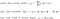(Via Eq 3.10, 3.15+) Demonstration of derivation of density matrices for pure and mixed states, pi is a classical probability with ∑p=1 .

The paper makes use of the trace operation (Tr) as well so I’ll expand on it quickly. When we have a combination of quantum substates, say state A which represents a qubit and state B which could be a second qubit or alternatively could represent the rest of the universe, by taking a trace in the B basis we’re effectively saying that we can choose to ignore the B basis and drop it altogether from our evaluation, which is a fortunate ability because I imagine nailing down the state space of the rest of the universe might be a challenge for most scientists. Looking at it from a linear algebra standpoint, a trace operation for a matrix can be derived from the sum of its diagonal elements.

Note that these density matrices are described in the paper as subject to the constraints of hermitian and positive semi-definite, let’s quickly define these terms. Hermitian matrices are square dimensions with nondiagnal entries such that the value of the complex scalar in row i and column j is equal to the complex conjugate of the value in row j and column i, where a complex conjugate just means that the imaginary part of the number is multiplied by -1. To be honest I’m not sure why this matters or how this constraint comes about so let’s just go with it. As for positive semi-definite, I think the easiest way to define this is the constraint that the eigenvalues of the matrix are positive. Of course this opens up a whole can of worms, now we have to address eigenvalues. Here’s my layman’s sketch (which I am basing heavily on the clear writeup in Wolfram mathworld): given a square matrix A, there exists a scalar eigen value λ and a corresponding (column) eigen vector X such that AX = λX. Thus λ is a kind of characteristic feature of a matrix, and it turns out there will be several such eigen values λi and corresponding eigen vectors Xi with quantity based on the size of the matrix. Further, it is possible to transform (e.g. rotate thought it’s dimensions) the basis of hermitian matrix A by applications derived from the set of eigenvectors to result in a matrix of same dimensions but whose values are all 0 save for the diagonals whose values are the set of eigen values λi, a process known as diagonalization.

When Witten describes the purification of a density matrix, this diagonalization property comes into play in the proof that we can create a process for incorporation of an orthogonal state space derived for a specific mixed state ρA such that when such unitary transformation is applied it transforms what started as a mixed state ρA to a pure state ψAB.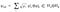Eq 3.13 — Demonstration of the purification of the state A by application of an orthogonal state B.

Before closing this section one further note on this Bra Ket notation, which I’ll include as kind of an aside and because it’s relevant and fundamental to many of my earlier writings on quantum computation even though it’s not directly addressed by Witten. For the special case of quantum computing with a qubit measured in the binary 0/1 basis (as opposed to say the +/- basis for the instance of another common basis) and thus a 2 dimensional Hilbert space, the inner product of the state descriptor Bra vector and the qubit measurement identifier vector Ket can be combined between multiple qubits using the tensor product operation for a joint state of multiple qubits such as we might get as we scale up our quantum computers. Here the Ket |0> refers to a single qubit measurement generating a 0, a |1> refers to a measurement generating a 1, with after combining two qubits for instance the Ket |00> indicates measurement on both qubits produces a 0, |01> indicating the first qubit measures a 0 and the second qubit a 1, and etc for |10> and |11>, with this combined Hilbert space dimensions of 2^n where n is the number of qubits. We can describe the state of a single qubit’s superposition as |ψ> = a|0> + b|1>, where a and b are complex scalars subject to the constraint |a|²+|b|² = 1. Combining multiple qubits thus entails: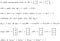Demonstration of deriving state space for a pair of qubits. Note that this can be further extended to additional qubits via tensor product, and the summed constraint to unity of the qubit states representing a sum of their respective probabilities for generating the corresponding measurements. Note that as we transition from a pure state to a mixed state’s density matrix (such as after incorporation of some channel of error to our state), the summed unity constraint =1 changes to <=1, and a value of zero would correspond to a fully decohered / maximally mixed state, this value will always shrink for real qubits with increasing time t with a rate based on the architecture, although its decline can be slowed by incorporating error correction.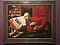St Jerome in His Study — from Workshop of Marinus van Reymerswaele

Now that we have the vocabulary to describe quantum states and their associated density matrices, we can progress to evaluating the informational entropy measure of these states. The quantum equivalent of the classical information theory’s Shannon entropy measure for a quantum state is called the von Neumann entropy, evaluated against a density matrix ρ. We incorporate the Trace operation into the definition to clarify that we are ignoring properties external to our basis of analysis. The paper’s comment that this S is manifestly invariant under a unitary transformation can be interpreted to mean that S won’t change with the application of a unitary transformation (such as for instance the application of quantum gates to a qubit (or collection thereof)), and we’ll find the same to be true when we get around to quantum conditional and relative entropy in section 3.4.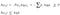(via Eq’s 3.17, 3.21, 3.22, 3.23) Demonstration of the von Neumann entropy S, where ρ is the density matrix under evaluation and pi is the classical probability associated with each of k constituent pure states ψi of that density. The positive constraint is derived by comparison of the summation form with the classical equivalent.

It’s an important point to consider that while the Shannon entropy was null (S=0) when the state was known with certainty, here the quantum equivalent von Neumann entropy is null (S=0) for a pure state ψ with rank 1 density matrix (i.e. pi = 1 for pure state ψi). That gives the lower bound of S, the upper bound of S corresponds to a perfectly mixed state between k constituent pure states such that the density matrix sees a constant classic probability for each as pi = 1/k for all pi.

Remember when we considered the classical Shannon entropy for the joint distribution of two distributions X and Y? Now we can look at a quantum equivalent for a pure state derived from tensor product of two pure states ψAB = ψA ⊗ ψB. The paper implies that we should be fascinated when we then compare the von Neumann entropies of each state ψA and ψB and find they are equal as SA = SB, although really this is only happening because for any pure state, including a composite pure state as well as those pure states from which it was derived, pi=1, so it’s not really that shocking of a result albeit it is unique to the quantum case.

This section closes by considering the Trace operation applied to a composite mixed state. I’ll discuss the trace operation further in my comments to section 3.5 so for now will just defer to Witten’s treatment.

The concavity feature is described for the combination of two density matrices ρ1 and ρ2, where the von Neumann entropy S is concave with respect to the mixing ratio t.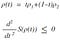Eq 3.34 — For the ensemble density matrix of two sub density matrices, the von Neumann entropy S is concave with respect to the mixing ratio t.

Recall that density matrices are statistical ensembles of pure states using classic probability ratios, derived from the sum of self tensor product matrix of each pure state multiplied by that state’s probability ρ = ∑pi*|ψi><ψi|. It follows then that a collective ensemble of two pure states can be developed simply by multiplying the first pure state by a mixing ratio t (with 0<=t<=1) and the second by (1-t) and then summing the two together as ρ(t) = tρ1 + (1-t)ρ2, which is what Witten demonstrates. The insight of this section comes into play when we consider the first and second order derivatives of the entropy corresponding to that ensemble density matrix with respect to t S(ρ(t)) , e.g. S’(ρ(t)), and S’’(ρ(t)), and it turns out after derivation that the second order derivative is negative for increasing t, S’’(ρ(t))<0, which in mathematic terms can be described as “concavity”.

Concavity and it’s counter convexity are fairly straight forward concepts that describe the sign of a second order derivative of some function. I find a handy way to picture these features graphically is that the graph for a convex function against some variable will curve up in one or both tails such as a happy smiling face, while a concave function will carry the frowning shape of someone who might be feeling a tad blue. The paper calls out that entropy can only increase under mixing, and thus there will be some mixing ratio 0<t<1 which maximizes the entropy S(ρ(t)). I expect that when ρ1=ρ2 and are pure states (of rank 1) then t can be any value 0<=t<=1 for maximum S, which I believe corresponds to the case where the second order derivative d2S(ρ(t))/dt2 = 0, the only exception to the concavity.

This section concludes by asking an interesting question. If we have a density matrix ρ which includes nondiagonal entries, and we drop from ρ those (non)diagonal matrix elements to be replaced by 0’s to derive what we’ll call ρD, what does the mixing function look like between these two versions ρ and ρD? As an aside this type of operation I believe corresponds to a non-unitary transformation such as might change a matrix’s set of eigen values, similar to a qubit experiencing some channel of error. As for the result, Witten uses this case to illustrate that dropping the off-diagonal part of a density matrix (in any basis) can only increase the entropy. Witten later offers an example for the physical realization of this diagnalization procedure in section 3.7.

In sections 2.2 and 2.3 we introduced conditional and relative entropy for classical information, here we explore the quantum equivalent, but first recall that in section 2.2 we demonstrated an entropy formula for joint distributions SXY. In the quantum world we have the two different types of joint distributions — those pure states joined via tensor product into a superposition, and alternatively a dense state consisting of a statistical ensemble of pure states — in both cases described by a density matrix. This is mostly speculation but I expect one of the indications of whether to expect a pure state ensemble or a dense matrix ensemble when joining a series of quantum states could involve the scale of address — for example two electrons interacting with each other might interact into a joint superposition pure state, while interacting features at the scale where environmental influence comes into play would interact as a statistical ensemble, the difference between nano scale and macro scale — this is just speculation though and I don’t consider myself an expert. In either case the entropy of the joint system will be as derived in 3.2, as a function of the constituent density matrices for the ensemble.

In 2.2 we derived for the classical case the conditional entropy for a bipartite system where Bob was trying to reconstruct a signal from Alice based only on his knowledge of her signal’s distribution, S(X|Y) which we found we could simplify to S(X|Y) = SXY — SY >= 0. In the quantum case we’ll use that same definition even though as Witten points out there’s not really a good quantum notion of conditional probabilities, and the fascinating point is that unlike for the classic case, in the quantum case S(A|B) negative values are allowed.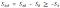(via eq 3.43, 3.48) — Quantum conditional entropy of two states A and B, where unlike the classical case SA|B is not bounded as nonnegative.

Recall that we discussed a purification process in section 3.1 whereby we could transform a dense ensemble to a pure state by conjoining with some specific orthogonal dense state, such that even though SB>0, as a pure state the purified SAB = 0. This proves that it is possible for SAB < SB, and it also corresponds to the boundary condition where SA|B = -SA.

Recall the classical property of mutual information which quantified the information about X that Bob gains from what he sees in signal Y. Just as in the case of conditional entropy, even though there isn’t a direct corollary for inferring information about one quantum state from another, we can still borrow the definition from the classical realm, and this time we even retain the nonnegative constraint (where I(A;B)=0 when ρAB factorizes, meaning ρAB=ρA⊗ρB).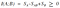(via eq’s 3.44, 3.45) — Quantum mutual information between two states.

Witten next addresses the question of why this quantum version of mutual information maintains the nonnegative constraint, and to do so he introduces another quantum analog to a previously discussed classical property, in this case relative entropy, S(A||B). Recall that we discussed relative entropy in section 2.3 and that it served as a way to measure the difference between a hypothesis distribution Q vs an actual distribution P, aka the Kullback-Liebler divergence. Witten first proves nonnegativity of this relative entropy, and then demonstrates that it can be derived using the same formula as mutual information, just as we did in the classical case.(via eq’s 3.49–3.54) — Use of the non negativity of quantum relative entropy to prove comparable boundedness of quantum mutual information.

I’ll close this section with a somewhat off-topic observation. As can be inferred from David Deutsch’s proof of universal quantum computation, once we have purified an arbitrary density matrix, I expect it would be possible to translate a pure state ψ1 to some arbitrary posterior pure state ψ2 of equivalent Hilbert space simply by the application of unitary transforms such as may be realized for a qubit using a universal set of quantum gates, with our only bound the constraints of these equations for conditional and relative quantum entropy.

Recall that the monotonicity of classical entropy was demonstrated by the comparison of the relative entropy (Kullback-Liebler divergence) between two distributions, such as may be evaluated in comparison of a hypothesis and actual distribution, and that the property can be described by the observance that the relative entropy between two multivariate distributions will be >= a comparable relative entropy of the same distributions after dropping one of the variables.Eq 2.28 — Restatement of the monotonicity of (classical) relative entropy, as observations of Y will only help our ability to infer differences between our hypothesis distribution Q(X,Y) and actual distribution P(X,Y).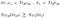(via eq’s 3.57, 3.58) The monotonicity of (quantum) relative entropy, where the trace operation is employed in the reduction of the Hilbert space for comparison.

In the quantum world, the trace operation (Tr) serves as the equivalent to this dropping a variable by reducing the Hilbert space under evaluation. For an example of a quantum computer, a trace operation could be realized by the measurement of a qubit causing the collapse of that portion of the collective superposition, or alternatively just by discarding the qubit such that no further interaction is realized in a fashion that can inform the evaluation.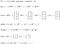Demonstration of a trace operation performed on a two-qubit computer’s state space. The “not equal” statement in the line with the trace operation was inferred from our discussion in section 3.1 — this is not related to the relative entropy narrative just a point that I found interesting. This is just speculation but my intuition is telling me that there is probably a case where Tr2|ψ12>= |ψ1> , which case I’m guessing is when for the orthogonal case |ψ1> ⊥ | ψ2> (such as we might get comparing the standard 0/1 basis with the +/- basis using terms familiar to those in quantum computer field) — for a definition of the +/- basis see my prior post “Quibbling Over Qubits”. (there’s just not a good mathematical notation (at least that I know of) to relate two sides of an equation as “might be equal to each other but might not”, something like ≤ or ≥, I was tempted to use “≠=“ but didn’t want to base a precise equation on speculation.)Note the relation for the set of a/b/c/d that could be inferred by relating the two unitary constraints from the trace demonstration above. This is not related to the relational entropy narrative just a point that I found interesting.

Jumping back to the monotonicity narrative, Witten then uses the result of monotonicity of (quantum) relative entropy coupled with the corresponding monotonicity of mutual information to derive a demonstration of what he has called the miraculous result of strong subadditivity in quantum information, along with a few equivalent statements, including some relating to entropy of combined systems and some related to conditional entropy of systems.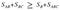Eq 3.65 — Strong subadditivity of quantum information.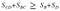Eq 3.66 — An equivalent statement to strong subadditivity of quantum information.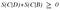Eq 3.67 — Per Witten, “a given qubit in C can be entangled with D, reducing SCD, or with B, reducing SBC, but not both.”

So what is it about strong subadditivity of quantum information that causes Witten to use a word like miraculous? This section opens with a comparison to classical probability, where although classical probability allows for joint multivariate distributions, there is no equivalent in quantum observables. Despite this disparity, many of the conventions of classical information theory extend into quantum information equivalents, albeit with a few adjustments such as the potential for negativity in conditional entropy.

3.6 Generalized Measurements

As this section addresses measurements, it’s probably good to figure out what is meant by the orthogonal hermitian projection operators πs for s from 1 to k, where k is the number of Hilbert dimensions. First from what I’ve been able to gather about projection operators, πs is a square matrix which wasn’t explicitly stated in the paper (although I think that might be implied by the use of the word “operator”). I found an improved description of the derivation of the measurement operator in Nielsen and Chuang’s text Quantum Computation and Quantum Information, section 2.2.3. Basically we have a set {πs } which is in the form of a horizontal row vector like a Bra vector <πs|. We can then apply an outer product between a corresponding Ket vector |πs> to derive the square matrix operator (recall that we can translate from a Bra to Ket vector using the conjugate-transpose operation).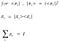Demonstration of the derivation of a square matrix projection operator from a set of measurement operators {πs}, subject to the constraint that the summation for s from 1 to k produces the identity matrix I.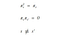(Via Eq 3.68) Further constraints on the measurement operator. The first here πs²=πs is a way of saying that no matter how many times we apply the operator the results will remain unchanged from the first application. The second here is a way of say that for all s πs is orthogonal to πs’. The third constraint is just a way of saying that each πs is distinct.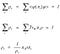(Via Eq’s 3.69–3.71) Here ps is the classical probability of a measurement generating new density matrix ρs, demonstrated for both a pure state ψ as well as a dense state ρ. (The trace operation is performed against the remaining dimensions of the Hilbert space). Recall that from our discussions in section 3.1 that the output of a Bra Ket inner product incorporating an intermediate square matrix has an output as a complex scalar, and I’m guessing it is the constraints on the projection operators from equation 3.68 that ensure the projection operation produces a result as a real scalar instead of complex and hence a valid classical probability.

As an aside, note that the specific projection operator will be a function of our basis of measurement. A quantum computer is generally built to distinguish between the superposition of two opposite states such as 0/1 or +/-. It is possible to build a kind of qubit that upon measurement distinguishes between >2 states (e.g. a 3 state qutrit), but I believe it has been demonstrated that the binary basis will generally have close to comparable computationally efficiency.

Turning back to the paper, Witten goes on to introduce linear operators Es for s:1-k which although carry some of the same formulas for application to a state, are not orthogonal projection operators but are derived from the application of some unitary transformation. This is a more general kind of quantum mechanical measurement which we’ll learn more about in the next section. These linear operators Es are called Kraus operators.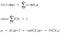Eq’s 3.72–3.73 — Derivation of the linear operators Es for s in 1-k from some unitary operator U, a more general kind of quantum measurement operator for arbitrary non-orthogonal projections such as we might employ with a qutrit.

Since we are talking about the measurement operation here, I’ll close by going on a brief tangent related to measurements and associated time-based evolution of our quantum states, which will be inspired from an illustration included in Roger Penrose’s recent work “Fashion, Faith, and Fantasy in the New Physics of the Universe”. In this case the below image depicts the two types of evolution, deterministic evolution over time per the Schrödinger equation for time based evolution of a quantum state, and probabilistic state collapse. One of the things that can be inferred here is that the time evolution has a positive slope in all cases for increasing t, where I believe the y axis is a somewhat abstract representation for Schrödinger evolution. I think this is comparable to what I’ve already touched on in that for a qubit superposition the time evolution will always trend to the direction of decoherence. I think an important lesson I am inferring here is that this trend to decoherence is a different operation than the measurement projection, which goes in a different direction. I am thus inferring that a qubit’s trend to decoherence is driven by the evolution from a pure state to an increasing mixed dense state, such that a fully decohered qubit will be maximally mixed, with an invertible density matrix ρ, a different result that a qubit’s post-measurement state. Although to be honest I’m kind of speculating here.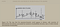This drawing has a lot of subtlety. (via “Fashion, Faith, and Fantasy in the New Physics of the Universe”)

This section addresses quantum channels for the evolution of a density matrix. Very simply a state ρ will evolve per the application of a unitary matrix U.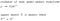(Via Eq 3.79) — Demonstration of the evolution of a density matrix ρ to ρ’ under unitary transform, where we provide a definition of unitary transform by a square unitary matrix U whose inverse is equal to its conjugate transpose.

This is the special case for evolution under a single unitary transformation (a set of Kraus operators with k=1) . For the more general case where we have a collection of unitary transforms, ρ will evolve via application of the set of k Kraus operators as we derived in the previous section.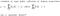Eq 3.83 — Evolution of density matrix ρ by application of a set of k Kraus operators (Es) aka a “quantum channel.”

In the derivation of this more general quantum channel via a set of Kraus operators, Witten provides a series of intermediate transformations in equations 3.80–3.82, which I won’t duplicate here in interest of leaving the good stuff where it belongs. But an important point is that after the preparations of a density matrix ρ and applications of Kraus operators to this intermediate state, a trace operation is performed to get to ρ’, which, when we then revisit the monotonicity of (quantum) relative entropy, is called out as the reason for this surprising result.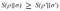Eq 3.84 — An equivalent statement to the monotonicity of (quantum) relative entropy (as previously discussed in section 3.5), where ρ and σ are density matrices subject to a comparable set of Kraus operators to derive ρ’ and σ’.

This section is then concluded by a series of exercises to illustrate properties of quantum channels:

1. A reminder of the purification operation, allowing us to map any arbitrary density matrix ρ to a pure state |ψ><ψ| via application of an appropriate set of Krauss operators.
2. A somewhat analogous case, where it is alternatively possible to map any arbitrary density matrix ρ to a maximally mixed density matrix via application of an appropriate set of Krauss operators.
3. A diagonalization operation to map any arbitrary density matrix ρ to ρD by dropping the non-diagonal elements of the matrix, with a demonstration of a physical realization of this operation offered.
4. Inferring the composite quantum channel comprised two joint quantum channels. (It would be really impressive if I worked this out here don’t ya think?)
5. Deriving Kraus operators to map to arbitrary states of reduced Hilbert space dimensions.
6. Deriving Kraus operators to map to arbitrary states of increased Hilbert space dimensions.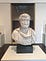Portrait Bust of a Bearded Man — 2nd — 3rd century CE

The discussion turns down some new territory in this section, and for the first time relates the quantum informational entropy value, aka a von Neumann entropy Sρ associated with a dense quantum state’s density matrix ρ, with a thermodynamic equivalent of entropy Sσ associated with that system’s thermal density matrix σ — such entropy as would be described by the second law of thermodynamics for instance. Although they are describing different phenomena, they are in the end both statistical measures derived from some distribution of probabilities. Some might even argue that the two measures share a greater equivalency, consider the “it from bit” hypothesis.

Witten here considers first the thermal density matrix σ at some temperature, and then draws of the relative entropy S(ρ||σ) for these two systems, along with the corresponding free energy F(ρ). The key finding is that assuming some quantum evolution of ρ->ρ’ under constant temperature such that σ’=σ, by making use of a statement similar to the monotonicity of relative entropy, the free energy can only be reduced by the application of a quantum channel.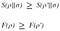Eq’s 3.90–3.91 For the application of an evolution per some quantum channel under constant temperature, the free energy can only go down (consistent with the second law of thermodynamics).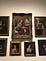Saint Cecilia — Simon Vouet (top center)

# 4 More On Quantum Information Theory

These closing passages are three topics that Witten found helpful in gaining insight about the meaning of quantum conditional entropy and quantum relative entropy (see section 3.4), with attention paid to the relevant implications of monotonicity of quantum relative entropy (see section 3.5) — and its close cousin, strong subadditivity.

Alice has in her possession a quantum state A0 which she wants to share with Bob. Due to the no-cloning theorem it is impossible to create an identical copy of an arbitrary unknown quantum state. However, quantum information allows for a teleportation of a state such that Bob can achieve a copy of the state provided that in the process Alice performs a measurement on her copy, causing her to lose her quantum information. (The teleportation term I suspect derives from the old Star Trek thought experiment — even though the Enterprise generates an exact copy of a crew member on the planet surface in the act of teleporting, the original version of that person is disintegrated in the process.) The teleportation is achieved by connecting Alice and Bob with two channels of communication: a quantum channel of entanglement between qubits A1 and B1 and a classical channel. Alice and Bob first generate an entanglement between a pair of qubits (one held by Alice and one by Bob) by preparing a Bell state ΨAB. (More on generating a Bell state here.)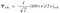Eq 4.1 — Alice and Bob’s Bell state, a shared entanglement between a qubit held by Alice (A1) and a qubit held by Bob (B1).

So keeping count, we now have three qubits, two held by Alice (A0 and A1), and one held by Bob (B1). A1 and B1 we’ve prepared in an entangled state (Eq 4.1), and the state of A0 that we want to teleport to Bob is in the unknown state A0 = α|0> + β|1>. Now as is possible for multi-qubit systems, in addition for allowing A1 to interact with B1, we can also at the same time allow A1 to interact with the target state A0 (Eq 4.2), thus facilitating a joint system of three qubits A0, A1, and B1 (Eq 4.3). Notice that the α and β from our A0 distribution are carried through into our joint distribution for A0A1B1 of Eq 4.3. And while we can’t measure the state A0 directly without causing a collapse of our target state, Alice can manipulate the pair A0A1 such that a measurement will collapse the joint distribution A0A1 into one of four possible two-qubit arrangements (Eq 4.2) while preserving the α and β distribution parameters of A0 amongst the joint interactions of A0A1B1 (Eq 4.3). So when we perform the measurement on A0A1, it tells us which of four possible two-qubit arrangements, which can be communicated to Bob in the classical channel allowing him to reconstruct a correction gate set (Eq 4.5 for the example of Eq 4.4) to his remaining distribution of B1 (post measurement on A0A1) to translate from ΨB1 to ΨA0, and thus after performing this manipulation Bob receives a copy of the state A0 “teleported” from Alice.

After first restating this approach in an alternate configuration (incorporating an addition state R maximally entangled with A0), Witten then proceeds to explore the relative entropy of the system components. Combining A0 and A1 into the joint system A and with B0 as B, we look at the conditional entropy S(A|B). Using the auxiliary system R in the derivation, there is an interesting statement made which I will point out because is novel idea to me: “A0 is maximally mixed, since it is maximally entangled with R”. After accounting for S=1 for maximimally mixed states and S=0 for pure states, it is shown that for this case with incorporation of R S(A|B) = 0, which turns out to be the limit allowed for successful teleportation, in and the more general case: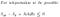(via Eq’s 4.8–4.9) Teleportation of quantum states are subject to this constraint on conditional entropy of Alice’s state A and Bob’s state B.

The discussion around justification for this constraint was a little over my head, but the fact of this limit better allows us to understand the parallel between classical and quantum conditional entropy. Using Witten’s words here “Remember that classically S(A|B) measures how many additional bits of information Alice has to send to Bob after he has already received B, so that he will have full knowledge of A”, and the analog for conditional entropy can be demonstrated by the sharing of (half of a pair of) entangled qubits to Bob, where for each of these entangled qubits Bob receives SB is increased by one. Thus if someone tells you they have a quantum conditional entropy S(A|B) = 1 and they want to teleport a state, tell them they’ll need first share at least one more pair of entangled qubits between Alice and Bob, and the more complex of Alice’s state A0 (and the higher of SAB) the more of these pairs they will need to share to offset.

This section opens by a restatement of some classical relative entropy principles and their quantum equivalents that were originally discussed in sections 2.3 and 3.4, where for sufficient number of observations N, we could estimate the probability that a hypothesis distribution was equivalent to an actual distribution.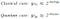Eq’s 4.19–4.20 — Restatement of relative entropy for both classical and quantum cases used to derive a probabilistic certainty of a hypothesis distribution matching an actual distribution, given number of observations N.

Recall the monotonicity of relative entropy (as discussed in section 3.5 and elsewhere) , Witten sites an external paper that presumably gives an upper bound on our ability to infer these probabilities from relative entropy, which result he then goes on to prove. In order to do so, he demonstrates a further equivalence between the quantum and classical cases of relative entropy. It turns out that in the act of making our observations on the quantum case, which are in effect mapping our quantum density matrices via partial trace onto classical probability distributions such that our ρ and σ distributions are replaced with corresponding classical distributions R and S. Using the monotonicity of relative entropy definition (Eq 4.25), and noting that for the resulting probabilistic derivations as we are rising the 2 to the negative power it will switch the direction of the > sign, we end up with this limit that the process of converting our density matrices to corresponding classical probability collections, the act of performing an additional quantum transformation in the translation means that we will have a higher degree of certainty post conversion, albeit one that will wash out as N gets sufficiently large.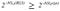Eq 4.26 — After converting our quantum distributions ρ and σ to corresponding classical sets R and S via application of partial trace operations, we end up with a higher certainty for validity of our hypothesis distribution, which advantage fades with increasing observations N.

Note that once the information is translated into classical space, it’s now possible to make copies, and so N observations can turn into N observations of N’ copies, which comes up later in the discussion although to be honest most of what follows here is over my head.

Of the recurring themes in this research, one of the most consistent has been the explorations of boundary conditions. In the fields of information theory — first in classical and then in quantum, what are the limits of our ability to transmit and to infer given the basis of transmission. More so, we have asked how things change now that we have entered the quantum world — where do the assumptions of classical information theory change in this less intuitive environment. In this the closing passage, the author asks these questions one more time.

When Claude Shannon laid the foundations for information theory, he did so by asking questions about information content of a signal, and what can be inferred about transmission density by inspecting of a signal’s contents, through which he derived the fundamental measure of entropy, a unit that was based on similar probabilistic elements to what had previously been used by physicists to analyze thermodynamic systems. Here as we have extended the basis of Shannon’s classical information theory to consider systems incorporating quantum information, we have found many analogues to those limits of classical information, albeit in a few cases some more surprising aspects of quantum information. It is fitting that Witten closes his paper by asking a question for quantum information very similar to what Shannon must have first asked himself for digital transmissions: how many bits of information can Alice send to Bob by sending him a quantum system X with a k-dimensional Hilbert space H? In the interest of leaving the good stuff where it belongs, I won’t walk through Witten’s derivation here. Suffice to say, it is demonstrated that the best Alice can do in the sharing of k orthogonal basis vectors is to share log k bits of information. This is the boundary of quantum information.

• Just to restate the obvious, this essay has been a partial summary and partial response to a recently published paper by the physicist Edward Witten, which pre-print is available on arXiv here: A Mini-Introduction To Information Theory [arXiv:1805.11965].
• All of the equations were either sourced from or derived from that work (with a few exceptions for the quantum computing demonstrations).
• The photos from this collection were taken during a recent road trip to Austin, TX — including photos of the collections of Bullock Texas State History Museum, Harry Ransom Center, and the Blanton Museum of Art.

Books that were referenced here or otherwise inspired this post:

(As an Amazon Associate I earn from qualifying purchases.)

Albums that were referenced here or otherwise inspired this post:

(As an Amazon Associate I earn from qualifying purchases.)

Hi, I’m an amateur blogger writing for fun. If you enjoyed or got some value from this post feel free to like, comment, or share. I can also be reached on linkedin for professional inquiries or twitter for personal.

Written by

## From the Diaries of John Henry

#### Essays and Explorations

Welcome to a place where words matter. On Medium, smart voices and original ideas take center stage - with no ads in sight. Watch
Follow all the topics you care about, and we’ll deliver the best stories for you to your homepage and inbox. Explore
Get unlimited access to the best stories on Medium — and support writers while you’re at it. Just \$5/month. Upgrade